Next: Rotational motion Up: Circular motion Previous: Worked example 7.4: Aerobatic

## Worked example 7.5: Ballistic pendulum

Question: A bullet of mass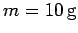strikes a pendulum bob of mass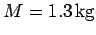horizontally with speed, and then becomes embedded in the bob. The bob is initially at rest, and is suspended by a stiff rod of length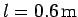and negligible mass. The bob is free to rotate in the vertical direction. What is the minimum value ofwhich causes the bob to execute a complete vertical circle? How does the answer change if the bob is suspended from a light flexible rod (of the same length), instead of a stiff rod?

Answer: When the bullet strikes the bob, and then sticks to it, the bullet and bob move off with a velocity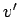which is given by momentum conservation: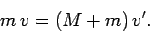Hence,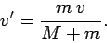Consider the case where the bob is suspended by a rigid rod. If the bob and bullet only just manage to execute a vertical loop, then their initial kinetic energy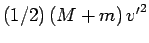must only just be sufficient to lift them from the bottom to the top of the loop--a distance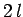. Hence, in this critical case, energy conservation yields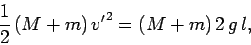which implies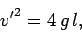or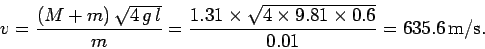Consider the case where the bob is suspended by a flexible rod. The velocity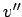of the bob and bullet at the top of the loop is obtained from energy conservation: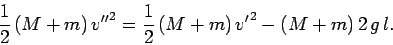If the bob and bullet only just manage to execute a vertical loop, then the tension in the rod is zero at the top of the loop. Hence, the acceleration due to gravitymust account exactly for the required acceleration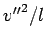towards the centre of the loop: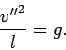It follows that, in this critical case,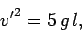or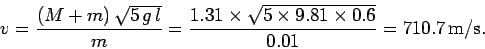Next: Rotational motion Up: Circular motion Previous: Worked example 7.4: Aerobatic
Richard Fitzpatrick 2006-02-02